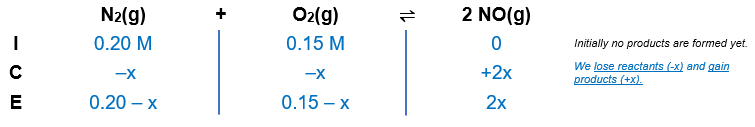# Problem: Even at high T, the formation of NO is not favored: N2(g) + O2(g) ⇌ 2NO(g)         Kc = 4.10×10−4 at 2000°C What is [NO] when a mixture of 0.20 mol of N 2(g) and 0.15 mol of O2(g) reach equilibrium in a 1.0-L container at 2000°C?

###### FREE Expert Solution

N2(g) + O2(g) ⇌ 2NO(g)         Kc = 4.10×10−4 at 2000°C

Step 1: Calculate the initial concentrations.

[N2] = 0.20 M

[O2] = 0.15 M

Step 2: Construct an ICE chart for the equilibrium reaction.Step 3: Calculate the change (x) in the reaction using the equilibrium constant.

N2(g) + O2(g) ⇌ 2NO(g)         Kc = 4.10×10−4

${\mathbf{K}}_{\mathbf{c}}\mathbf{=}\frac{{\mathbf{\left[}\mathbf{NO}\mathbf{\right]}}^{\mathbf{2}}}{\mathbf{\left[}{\mathbf{N}}_{\mathbf{2}}\mathbf{\right]}\mathbf{\left[}{\mathbf{O}}_{\mathbf{2}}\mathbf{\right]}}\phantom{\rule{0ex}{0ex}}\mathbf{4}\mathbf{.}\mathbf{10}\mathbf{×}{\mathbf{10}}^{\mathbf{-}\mathbf{4}}\mathbf{=}\frac{{\mathbf{\left(}\mathbf{2}\mathbf{x}\mathbf{\right)}}^{\mathbf{2}}}{\mathbf{\left(}\mathbf{0}\mathbf{.}\mathbf{20}\mathbf{-}\mathbf{x}\mathbf{\right)}\mathbf{\left(}\mathbf{0}\mathbf{.}\mathbf{15}\mathbf{-}\mathbf{x}\mathbf{\right)}}\phantom{\rule{0ex}{0ex}}\mathbf{4}\mathbf{.}\mathbf{10}\mathbf{×}{\mathbf{10}}^{\mathbf{-}\mathbf{4}}\mathbf{\left(}\mathbf{0}\mathbf{.}\mathbf{03}\mathbf{-}\mathbf{0}\mathbf{.}\mathbf{35}\mathbf{x}\mathbf{+}{\mathbf{x}}^{\mathbf{2}}\mathbf{\right)}\mathbf{=}\frac{\mathbf{4}{\mathbf{x}}^{\mathbf{2}}}{\overline{)\mathbf{0}\mathbf{.}\mathbf{03}\mathbf{-}\mathbf{0}\mathbf{.}\mathbf{35}\mathbf{x}\mathbf{+}{\mathbf{x}}^{\mathbf{2}}}}\mathbf{\left(}\overline{)\mathbf{0}\mathbf{.}\mathbf{03}\mathbf{-}\mathbf{0}\mathbf{.}\mathbf{35}\mathbf{x}\mathbf{+}{\mathbf{x}}^{\mathbf{2}}}\mathbf{\right)}\phantom{\rule{0ex}{0ex}}\mathbf{1}\mathbf{.}\mathbf{23}\mathbf{×}{\mathbf{10}}^{\mathbf{-}\mathbf{5}}\mathbf{-}\mathbf{\left(}\mathbf{1}\mathbf{.}\mathbf{435}\mathbf{×}{\mathbf{10}}^{\mathbf{-}\mathbf{4}}\mathbf{\right)}\mathbf{x}\mathbf{+}\mathbf{\left(}\mathbf{4}\mathbf{.}\mathbf{10}\mathbf{×}{\mathbf{10}}^{\mathbf{-}\mathbf{4}}\mathbf{\right)}{\mathbf{x}}^{\mathbf{2}}\mathbf{=}\mathbf{4}{\mathbf{x}}^{\mathbf{2}}\phantom{\rule{0ex}{0ex}}\overline{)\mathbf{0}\mathbf{=}\mathbf{3}\mathbf{.}\mathbf{99959}{\mathbf{x}}^{\mathbf{2}}\mathbf{+}\mathbf{\left(}\mathbf{1}\mathbf{.}\mathbf{435}\mathbf{×}{\mathbf{10}}^{\mathbf{-}\mathbf{4}}\mathbf{\right)}\mathbf{x}\mathbf{-}\mathbf{1}\mathbf{.}\mathbf{23}\mathbf{×}{\mathbf{10}}^{\mathbf{-}\mathbf{5}}}$

Now, we will use the quadratic formula to solve for x. The quadratic formula is:

$\overline{){\mathbf{x}}{\mathbf{=}}\frac{\mathbf{-}\mathbf{b}\mathbf{±}\sqrt{{\mathbf{b}}^{\mathbf{2}}\mathbf{-}\mathbf{4}\mathbf{a}\mathbf{c}}}{\mathbf{2}\mathbf{a}}}$

Solving for x:

$\mathbf{x}\mathbf{=}\frac{\mathbf{-}\mathbf{\left(}\mathbf{1}\mathbf{.}\mathbf{435}\mathbf{×}{\mathbf{10}}^{\mathbf{-}\mathbf{4}}\mathbf{\right)}\mathbf{±}\sqrt{\mathbf{\left(}\mathbf{1}\mathbf{.}\mathbf{435}\mathbf{×}{\mathbf{10}}^{\mathbf{-}\mathbf{4}}{\mathbf{\right)}}^{\mathbf{2}}\mathbf{-}\mathbf{4}\mathbf{\left(}\mathbf{3}\mathbf{.}\mathbf{99959}\mathbf{\right)}\mathbf{\left(}\mathbf{-}\mathbf{1}\mathbf{.}\mathbf{23}\mathbf{×}{\mathbf{10}}^{\mathbf{-}\mathbf{5}}\mathbf{\right)}}}{\mathbf{2}\mathbf{\left(}\mathbf{3}\mathbf{.}\mathbf{99959}\mathbf{\right)}}\phantom{\rule{0ex}{0ex}}\mathbf{x}\mathbf{=}\frac{\mathbf{-}\mathbf{\left(}\mathbf{1}\mathbf{.}\mathbf{435}\mathbf{×}{\mathbf{10}}^{\mathbf{-}\mathbf{4}}\mathbf{\right)}\mathbf{±}\sqrt{\mathbf{2}\mathbf{.}\mathbf{059225}\mathbf{×}{\mathbf{10}}^{\mathbf{-}\mathbf{8}}\mathbf{+}\mathbf{1}\mathbf{.}\mathbf{9678}\mathbf{×}{\mathbf{10}}^{\mathbf{-}\mathbf{4}}}}{\mathbf{7}\mathbf{.}\mathbf{99918}}\phantom{\rule{0ex}{0ex}}\mathbf{x}\mathbf{=}\frac{\mathbf{-}\mathbf{\left(}\mathbf{1}\mathbf{.}\mathbf{435}\mathbf{×}{\mathbf{10}}^{\mathbf{-}\mathbf{4}}\mathbf{\right)}\mathbf{±}\sqrt{\mathbf{1}\mathbf{.}\mathbf{968}\mathbf{×}{\mathbf{10}}^{\mathbf{-}\mathbf{4}}}}{\mathbf{7}\mathbf{.}\mathbf{99918}}\phantom{\rule{0ex}{0ex}}\mathbf{x}\mathbf{=}\frac{\mathbf{-}\mathbf{\left(}\mathbf{1}\mathbf{.}\mathbf{435}\mathbf{×}{\mathbf{10}}^{\mathbf{-}\mathbf{4}}\mathbf{\right)}\mathbf{±}\mathbf{0}\mathbf{.}\mathbf{014}}{\mathbf{7}\mathbf{.}\mathbf{99918}}$

The calculation then splits into two ways:

97% (194 ratings)###### Problem Details

Even at high T, the formation of NO is not favored:

N2(g) + O2(g) ⇌ 2NO(g)         Kc = 4.10×10−4 at 2000°C

What is [NO] when a mixture of 0.20 mol of N 2(g) and 0.15 mol of O2(g) reach equilibrium in a 1.0-L container at 2000°C?

Frequently Asked Questions

What scientific concept do you need to know in order to solve this problem?

Our tutors have indicated that to solve this problem you will need to apply the ICE Chart concept. You can view video lessons to learn ICE Chart. Or if you need more ICE Chart practice, you can also practice ICE Chart practice problems.

What professor is this problem relevant for?

Based on our data, we think this problem is relevant for Professor Wink's class at UIC.

What textbook is this problem found in?

Our data indicates that this problem or a close variation was asked in Chemistry: The Molecular Nature of Matter and Change - Silberberg 8th Edition. You can also practice Chemistry: The Molecular Nature of Matter and Change - Silberberg 8th Edition practice problems.